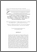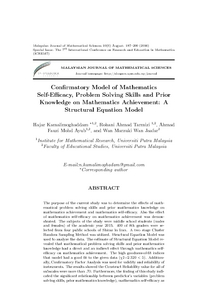# Confirmatory model of mathematics self-efficacy, problem solving skills and prior knowledge on mathematics achievement: a structural equation model

## Citation

Kamalimoghaddam, Hajar and Ahmad Tarmizi, Rohani and Mohd Ayub, Ahmad Fauzi and Wan Jaafar, Wan Marzuki (2016) Confirmatory model of mathematics self-efficacy, problem solving skills and prior knowledge on mathematics achievement: a structural equation model. Malaysian Journal of Mathematical Sciences, 10 (spec. Aug.). pp. 187-200. ISSN 1823-8343; ESSN: 2289-750X

## Abstract

The purpose of the current study was to determine the effects of mathematical problem solving skills and prior mathematics knowledge on mathematics achievement and mathematics self-efficacy. Also the effect of mathematics self-efficacy on mathematics achievement was demonstrated. The subjects of the study were middle school students (males and females) of the academic year 2013. 400 of 8th graders were selected from four public schools of Shiraz in Iran. A two stage Cluster Random Sampling Method was utilized. Structural Equation Model was used to analyze the data. The estimate of Structural Equation Model revealed that mathematical problem solving skills and prior mathematics knowledge had a direct and an indirect effect through mathematics self-efficacy on mathematics achievement. The high goodness-of-fit indices that model had a good fit to the given data (χ2=2.520 < 5). Additionally, Confirmatory Factor Analysis was used for validity and reliability of instruments. The results showed the Construct Reliability value for all of subscales were more than .70. Furthermore, the finding of this study indicated the significant relationship between predictor’s variables (problem solving skills, prior mathematics knowledge), mathematics self-efficacy as mediator, and mathematics achievement as criterion variable. The findings supported the hypothesized role of self-efficacy in Bandura’s (1986) social cognitive theory and Schoenfeld’s (1985) mathematical problem solving theory. This study hopes to accomplish a perfect framework for educators, students, and the Ministry of Education. The obtained results of the current study will bring advantages to the researchers and educators.Preview
PDF
14. Hajar.pdfView Item